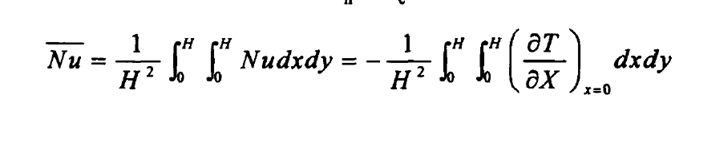# openfoam里怎么写求Nu面平均的方程

•这个方程里面对面进行积分怎么表示

• 二维的话，第一个等号后面就是$\frac{1}{S}\cdot Nu\cdot S, S=H^2$。三维的话第一个等号后面就是$\frac{1}{V}\cdot Nu\cdot V, V=H^3$。在OpenFOAM里面就是体平均，差不多是

forAll(Nu, cell)
{
test += Nu*mesh.V();
}
test /= V;


• @东岳 谢谢 岳老师